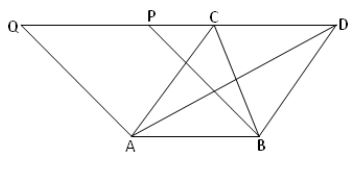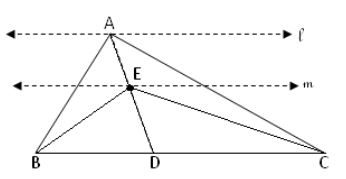# Triangle on Same Base and between Same Parallels Theorem – Solved Examples

When two triangles lie on the same base and between the same parallels, then those triangles measure equal area. Check the proof for Triangles on the same base and between the same parallel are equal in Area. Get the relationship between the areas of triangles having the same base in the following sections. For the reference of students, we have also included the solved examples on Triangle on the Same Base and between Same Parallels.

## Proof for Area of Triangles are Equal Having the Same Base

Get a detailed explanation for how the areas of triangles are equal when their bases are the same and between the same parallels.

In the following figure, ∆ABD and ∆DEF having equal base length and between the same parallels BF and AD.In this case, Area of △ ABC = Area of △ DEF

To prove that Triangle on the Same Base and between Same Parallels have the same area, follow these instructions.

Let us take △ ABC and △ ABD on the same base AB and between the same parallels AB and CD.Draw a parallelogram ABPQ with AB base and lying between the same parallels AB and Q.

The relationship between the area of the triangle and parallelogram says that when they lie on the same base and between the same parallels, then the triangle is half of the parallelogram area.

So, Area of ∆ ABC = 1/2 x Area of parallelogram ABPQ

In the same way, Area of ∆ ABD = 1/2 x Area of parallelogram ABPQ

Therefore, Area of ∆ ABC = Area of ∆ ABD.

Hence proved.

### Questions on Triangles on the Same Base and between Same Parallels

Example 1.

AD is the median of ∆ABC and ∆ADC. E is any point on AD. Show that area of ∆ABE = area of ∆ACE.

Solution:Here, AD is the median for △ ABC.

So, BD = CD

In the above figure, △ ABD and △ ADC are having equal bases BD and CD and they are between the same parallels BC and U.

So, Area of △ ADC = Area of △ ABD —– (i)

Therefore, Ed is the median for △ BCE

Now, BDE and CDE are having the equal base length BD = CD and between the same paralleks BC and m, then

Area of △ CDE = Area of △ BDE —— (ii)

Subtracting equation (i) and equation (ii), we get

Area of △ ADC – Area of △ CDE = Area of ∆ABD – Area of ∆BED

Area of △ ABE = Area of △ ACE

Hence, shown.

Example 2.

Prove that the medians of the triangle divide it into two triangles having equal area?

Solution:AD is the median of the ∆ BCA and AE is the altitude of ∆ BCA and also ∆ ADC.

And AE is perpendicular to BC

Here, AD is the median of ∆ ABC

Therefore, BD = CD

On multiplying both sides of the equation by AE, we get

BD x AE = CD x AE

Again multiply both sides by 1/2

1/2 x BD x AE = 1/2 x CD x AE

Area of ∆ABD = Area of ∆ADC

Hence shown.

Scroll to Top